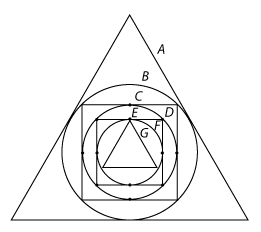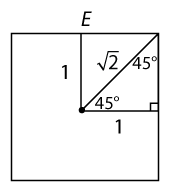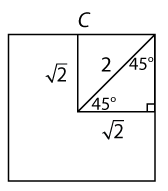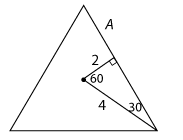# Manhattan GMAT Challenge Problem of the Week – 21 Dec 2010

by Manhattan Prep, Dec 21, 2010

Here is a new Challenge Problem! If you want to win prizes, try entering our Challenge Problem Showdown. The more people enter our challenge, the better the prizes.

## Question

Within equilateral triangle A, circle B is inscribed; within circle B, square C is inscribed; within square C, circle D is inscribed; within circle D, square E is inscribed; within square E, circle F is inscribed; finally, within circle F, equilateral triangle G is inscribed.

What is the ratio of the area of triangle A to the area of triangle G?

(A) 4 2 : 1

(B) 8 : 1

(C) 8 2 : 1

(D) 16 : 1

(E) 16 2 : 1

The first trick is to draw this complicated picture correctly!Next, work your way from the inside out. Pick the radius of circle F as 1. Notice that this is the distance from the center of triangle G to a vertex of G. For now, dont worry about finding the area of triangle Gwell see a shortcut later.

Now, what is the radius of the next circle out, circle D? 1 is the distance from the center of square E to the center of any side of that square. So we can draw a 45-45-90 triangle and find that the half-diagonal of square E is 2. This is also the radius of circle D.Applying the same reasoning, we can see that 2 is the distance from the center of square C to the center of any side of that square. We can draw another 45-45-90 triangle and find that the half-diagonal of square C is 2. This is also the radius of circle B.Finally, we can draw a 30-60-90 triangle within equilateral triangle A and see that the distance from the center to any vertex of triangle A is 4.Now, rather than calculate each area and divide, we can use a huge shortcut. The distance from center to vertex for triangle G was 1; the same distance for triangle A is 4. Since these two triangles are similar, this means that every distance ratio for the two triangles will be 4 : 1. For instance, their side lengths will be in a 4 : 1 ratio. And since areas are squares of distances, the ratio of areas will be [pmath]4^2[/pmath] to [pmath]1^2[/pmath], or 16 : 1.